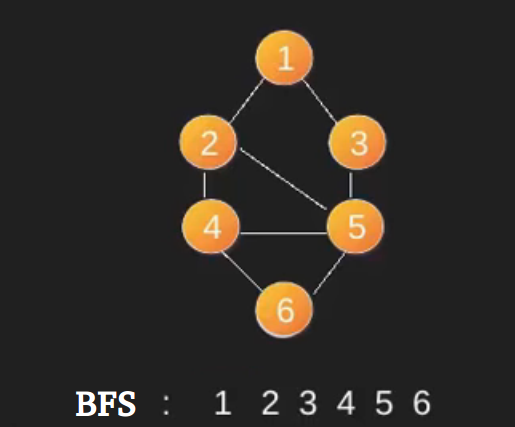# BFS using STL for competitive coding

A STL based simple implementation of BFS using queue and vector in STL. The adjacency list is represented using vectors of vector.

```In BFS, we start with a node.
1) Create a queue and enqueue source into it.
Mark source as visited.
2) While queue is not empty, do following
a) Dequeue a vertex from queue. Let this
be f.
b) Print f
c) Enqueue all not yet visited adjacent
of f and mark them visited.
```

Below is an example BFS starting from source vertex 1. Note that there can be multple BFSs possible for a graph (even from a particular vertex).For more details of BFS, refer this post .

The code here is simplified such that it could be used in competitive coding.

 `// A Quick implementation of BFS using ` `// vectors and queue ` `#include ` `#define pb push_back ` ` `  `using` `namespace` `std; ` ` `  `vector<``bool``> v; ` `vector > g; ` ` `  `void` `edge(``int` `a, ``int` `b) ` `{ ` `    ``g[a].pb(b); ` ` `  `    ``// for undirected graph add this line ` `    ``// g[b].pb(a); ` `} ` ` `  `void` `bfs(``int` `u) ` `{ ` `    ``queue<``int``> q; ` ` `  `    ``q.push(u); ` `    ``v[u] = ``true``; ` ` `  `    ``while` `(!q.empty()) { ` ` `  `        ``int` `f = q.front(); ` `        ``q.pop(); ` ` `  `        ``cout << f << ``" "``; ` ` `  `        ``// Enqueue all adjacent of f and mark them visited  ` `        ``for` `(``auto` `i = g[f].begin(); i != g[f].end(); i++) { ` `            ``if` `(!v[*i]) { ` `                ``q.push(*i); ` `                ``v[*i] = ``true``; ` `            ``} ` `        ``} ` `    ``} ` `} ` ` `  `// Driver code ` `int` `main() ` `{ ` `    ``int` `n, e; ` `    ``cin >> n >> e; ` ` `  `    ``v.assign(n, ``false``); ` `    ``g.assign(n, vector<``int``>()); ` ` `  `    ``int` `a, b; ` `    ``for` `(``int` `i = 0; i < e; i++) { ` `        ``cin >> a >> b; ` `        ``edge(a, b); ` `    ``} ` ` `  `    ``for` `(``int` `i = 0; i < n; i++) { ` `        ``if` `(!v[i]) ` `            ``bfs(i); ` `    ``} ` ` `  `    ``return` `0; ` `} `

```Input:
8 10
0 1
0 2
0 3
0 4
1 5
2 5
3 6
4 6
5 7
6 7

Output:
0 1 2 3 4 5 6 7
```

This article is contributed by Nikhil Mahendran. If you like GeeksforGeeks and would like to contribute, you can also write an article using contribute.geeksforgeeks.org or mail your article to contribute@geeksforgeeks.org. See your article appearing on the GeeksforGeeks main page and help other Geeks.My Personal Notes arrow_drop_up
Article Tags :
Practice Tags :

24

Please write to us at contribute@geeksforgeeks.org to report any issue with the above content.